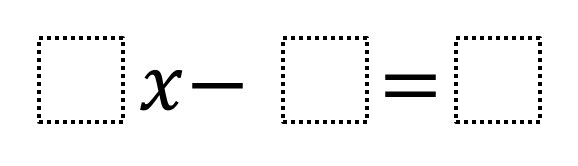# Two-Step Equations 2

Directions: Using the digits 1 to 9 at most one time each, fill in the boxes to find the largest (or smallest) possible values for x.### Hint

Where do you want to put the 9? Where should the 1 go or NOT go?

1x – 9 = 8 and 1x – 8 = 9 both have a solution of 17.
9x – 1 = 2 and 9x – 2 = 1 both have a solution of 1/3.

Source: Chase Orton and Mark Goldstein

## Similar Triangles And Slope

Directions: The three triangles on the line are similar. Using the digits 0 to 9 …

1.••